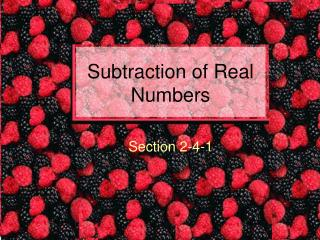DownloadDownload PresentationSubtraction of Real Numbers

Subtraction of Real Numbers

Télécharger la présentationSubtraction of Real Numbers

- - - - - - - - - - - - - - - - - - - - - - - - - - - E N D - - - - - - - - - - - - - - - - - - - - - - - - - - -
Presentation Transcript

1. Subtraction of Real Numbers Section 2-4-1

2. Rule: To subtract ADD the OPPOSITE. • This sounds easy. • Think about a problem 3 – 6. • The operation in this problem is subtraction. • The 6 is positive.

3. To follow the rule • Change the subtraction operation to addition • and the positive 6 to a negative 6. • The result is 3 +(-6). • Now follow the addition rules to get a result of –3.

4. 3 - 6 • It may be easier to think UP 3 DOWN 6 from the original 3-6 to arrive at –3.

5. Minus • The word minus can be used as a verb or an adjective in an equation. • It can mean to subtract or denote a negative number.

6. Example: -5.6 - (-3) • ADD the OPPOSITE –5.6+(3) = -2.6 • Notice the subtraction operation changed to addition, and • the opposite of –3 becomes 3.

7. 3.2 – 2.5 • ADD the OPPOSITE • –3.2 + (-2.5) =-5.7 • The – in front of the 2.5 is a verb. • It is a subtraction and this is what is changed to addition. • The – in front of the 3.2 is an adjective. It tells what kind of number 3.2 is.

8. UP and Down • An easy way to do these problems without writing down the new addition problem is think “UP” and “DOWN” at the appropriate time.

9. -2/3 –1/4 • Think: Down 2/3 down 1/4 more. • Get a common denominator. • 8/12 down and 3/12 down is –11/12.

10. 3-8 • Think: UP 3 then Down 8 for 5 in the hole. • 3-8 = -5 • -81-12 • Think Down 81 then Down 12 more. • –81 –12 = -93.

11. Two Negatives • When two negative are next to each other, you must write the new addition problem on paper. • 5-(-3) • ADD the OPPOSITE 5 + (+3) • Notice the use of the rule. • 5-(-3) = 5+3 = 8

12. -8 –(-4) • ADD the OPPOSITE • –8 + (+4). • Now think Down 8 then up 4. • -8-(-4) = -8+4 = -4

13. Double negatives • When double negatives are in long lines of addition and subtraction, • you must write the new addition. • However, you only need to change the double negative.

14. Example: -7-9-(-4)-3+9-(-4) • Changes on paper to –7-9+4-3+9+4. • Then think DOWN 7, Down 9 more, then UP 4, Down 3, UP 9, and UP 4. • The result is –2.